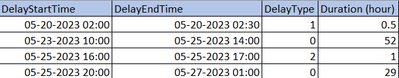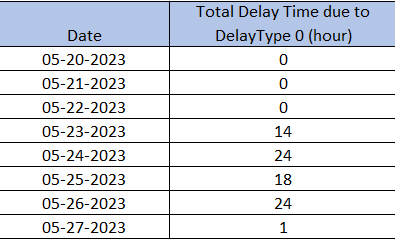cancel
Showing results for
Did you mean:New Member

## Help with calculating specific delay time per day

Hi, I am trying to make a report that would give the delay time per day (which would give me the run time per day to get approximate TPH). An example delay data table is formatted as shown below.Since I have to calculate tons per hour for every day using another table with production information, I created a Calendar table (just a one-column table with only the dates using the CALENDAR() function) that is connected with both the delay table and production table.

However, I am struggling to calculate the number of hours of delay time per day with DelayType 0 as a column in the calendar table with the format shown below using the previous delay table as an example.I tried making a calculated column using DATEDIFF() but I am struggling to get only the entries with DelayType of 0 as well as how to deal with delays that span for multiple days (For example, 05/26/2023 falls within the 4th entry of the delay table so the total delay time for that day would be 24 hours).

I would appreciate any help or guidance on what I can do to get the final formatted Calendar table. Thank you.

1 ACCEPTED SOLUTIONCommunity Support

According to your screenshot, I think you don't need to create a relationship between Calendar Table and Delay Table, due to datetime type column in Delay table and date type column in Calendar.

You can try this code to create a measure.

``````Total Delay Time due to DelayType 0 (hour) =
VAR _CROSSJOIN =
FILTER (
GENERATE (  ALLSELECTED('Delay Table') , CALCULATETABLE(VALUES ( 'Calendar'[Date] ),ALLSELECTED('Calendar')) ),
'Delay Table'[DelayType] = 0
&& DATEVALUE ( 'Delay Table'[DelayStartTime] ) <= 'Calendar'[Date]
&& DATEVALUE ( 'Delay Table'[DelayEndTime] ) >= 'Calendar'[Date]
)
_CROSSJOIN,
"Duration",
VAR _MIN =
MINX (
FILTER (
_CROSSJOIN,
AND (
'Delay Table'[DelayStartTime] = EARLIER ( 'Delay Table'[DelayStartTime] ),
'Delay Table'[DelayEndTime] = EARLIER ( 'Delay Table'[DelayEndTime] )
)
),
[Date]
)
VAR _MAX =
MAXX (
FILTER (
_CROSSJOIN,
AND (
'Delay Table'[DelayStartTime] = EARLIER ( 'Delay Table'[DelayStartTime] ),
'Delay Table'[DelayEndTime] = EARLIER ( 'Delay Table'[DelayEndTime] )
)
),
[Date]
)
RETURN
SWITCH (
TRUE (),
[Date] = _MIN
&& [Date] = _MAX, [Duration (hour)],
[Date] <> _MIN
&& [Date] <> _MAX, 24,
[Date] = _MIN
&& [Date] <> _MAX,
DATEDIFF ( [DelayStartTime], [Date] + 1, MINUTE ) / 60,
[Date] <> _MIN
&& [Date] = _MAX, DATEDIFF ( [Date], [DelayEndTime], MINUTE ) / 60
)
)
RETURN

Result is as below.Best Regards,
Rico Zhou

If this post helps, then please consider Accept it as the solution to help the other members find it more quickly.

2 REPLIES 2Community Support

According to your screenshot, I think you don't need to create a relationship between Calendar Table and Delay Table, due to datetime type column in Delay table and date type column in Calendar.

You can try this code to create a measure.

``````Total Delay Time due to DelayType 0 (hour) =
VAR _CROSSJOIN =
FILTER (
GENERATE (  ALLSELECTED('Delay Table') , CALCULATETABLE(VALUES ( 'Calendar'[Date] ),ALLSELECTED('Calendar')) ),
'Delay Table'[DelayType] = 0
&& DATEVALUE ( 'Delay Table'[DelayStartTime] ) <= 'Calendar'[Date]
&& DATEVALUE ( 'Delay Table'[DelayEndTime] ) >= 'Calendar'[Date]
)
_CROSSJOIN,
"Duration",
VAR _MIN =
MINX (
FILTER (
_CROSSJOIN,
AND (
'Delay Table'[DelayStartTime] = EARLIER ( 'Delay Table'[DelayStartTime] ),
'Delay Table'[DelayEndTime] = EARLIER ( 'Delay Table'[DelayEndTime] )
)
),
[Date]
)
VAR _MAX =
MAXX (
FILTER (
_CROSSJOIN,
AND (
'Delay Table'[DelayStartTime] = EARLIER ( 'Delay Table'[DelayStartTime] ),
'Delay Table'[DelayEndTime] = EARLIER ( 'Delay Table'[DelayEndTime] )
)
),
[Date]
)
RETURN
SWITCH (
TRUE (),
[Date] = _MIN
&& [Date] = _MAX, [Duration (hour)],
[Date] <> _MIN
&& [Date] <> _MAX, 24,
[Date] = _MIN
&& [Date] <> _MAX,
DATEDIFF ( [DelayStartTime], [Date] + 1, MINUTE ) / 60,
[Date] <> _MIN
&& [Date] = _MAX, DATEDIFF ( [Date], [DelayEndTime], MINUTE ) / 60
)
)
RETURN

Result is as below.Best Regards,
Rico Zhou

If this post helps, then please consider Accept it as the solution to help the other members find it more quickly.Super User

Total Delay Time due to DelayType 0 (hour) =
SUMX (
FILTER (
CROSSJOIN ( ALLSELECTED ( 'Delay Table' ), VALUES ( 'Calendar'[Date] ) ),
'Delay Table'[Delay Type] = 0
&& 'Delay Table'[DelayStartTime] <= 'Calendar'[Date]
&& 'Delay Table'[DelayEndTime] >= 'Calendar'[Date]
),
DATEDIFF (
MAX ( 'Delay Table'[DelayStartTime], 'Calendar'[Date] ),
MIN ( 'Delay Table'[DelayEndTime], 'Calendar'[Date] ),
MINUTE
) / 60
)